# One Hot Encoding of datasets in Python

Sometimes in datasets, we encounter columns that contain numbers of no specific order of preference. The data in the column usually denotes a category or value of the category and also when the data in the column is label encoded. This confuses the machine learning model, to avoid this the data in the column should be One Hot encoded.

## One Hot Encoding –

It refers to splitting the column which contains numerical categorical data to many columns depending on the number of categories present in that column. Each column contains “0” or “1” corresponding to which column it has been placed.

For example :

Consider the data where fruits and their corresponding categorical value and prices are given.

FRUIT CATEGORICAL VALUE OF FRUIT PRICE
apple 1 5
mango 2 10
apple 1 15
orange 3 20

The output after one hot encoding the data is given as follows,

APPLE MANGO ORANGE PRICE
1 0 0 5
0 1 0 10
1 0 0 15
0 0 1 20

Below is the Implementation in Python –

Example 1:

The following example is the data of zones and credit scores of customers, the zone is a categorical value which needs to be one hot encoded.

 `# Program for demonstration of one hot encoding ` `# import libraries ` `import` `numpy as np ` `import` `pandas as pd ` `# import the data required ` `data ``=` `pd.read_csv(r``"../../onehotenc_data.csv"``) ` `print``(data) `

Output: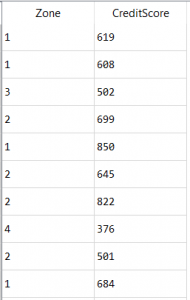To one hot encode the zone column –

 `# importing one hot encoder from sklearn ` `# There are changes in OneHotEncoder class ` `from` `sklearn.preprocessing ``import` `OneHotEncoder ` `from` `sklearn.compose ``import` `ColumnTransformer ` `# creating one hot encoder object with categorical feature 0 ` `# indicating the first column ` `columnTransformer ``=` `ColumnTransformer([(``'encoder'``, ` `OneHotEncoder(), ` `[``0``])], ` `remainder``=``'passthrough'``) ` `data ``=` `np.array(columnTransformer.fit_transform(data), dtype ``=` `np.``str``) `

Output: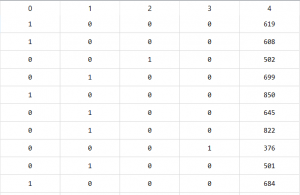The output contains 5 columns, one column for the price, and the remaining 4 columns representing the 4 zones.

Example 2:

One hot encoder only takes numerical categorical values, hence any value of string type should be label encoded before one-hot encoded.
The below example has the data of geography and gender of the customers which has to be label encoded first.

 `# importing libraries ` `import` `numpy as np ` `import` `pandas as pds ` `# After importing the required data ` `print``(data) `

Output: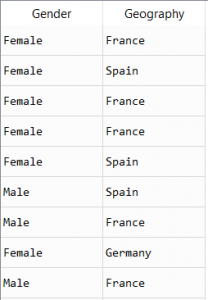Label encoding the data –

 `# label encoding the data ` `from` `sklearn.preprocessing ``import` `LabelEncoder ` `le ``=` `LabelEncoder() ` `data[``'Gender'``]``=` `le.fit_transform(data[``'Gender'``]) ` `data[``'Geography'``]``=` `le.fit_transform(data[``'Geography'``]) `

Output: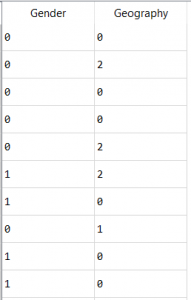One Hot Encoding Gender and Geography Columns –

 `# importing one hot encoder from sklearn ` `from` `sklearn.preprocessing ``import` `OneHotEncoder ` `# creating one hot encoder object by default ` `# entire data passed is one hot encoded ` `onehotencoder ``=` `OneHotEncoder() ` `data ``=` `np.array(columnTransformer.fit_transform(data), dtype ``=` `np.``str``) `

Output: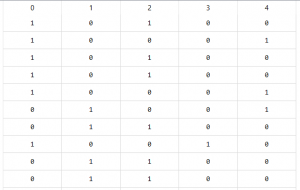The output contains 5 columns, 2 columns representing the gender, male and female, and the remaining 3 columns representing the countries France, Germany, and Spain.

Note :

1. The one hot encoder does not accept 1-dimensional array or a pandas series, the input should always be 2 Dimensional.
2. The data passed to the encoder should not contain strings.

# Special 95% discount

## 2000+ Applied Machine Learning & Data Science Recipes

### Portfolio Projects for Aspiring Data Scientists: Tabular Text & Image Data Analytics as well as Time Series Forecasting in Python & R## Two Machine Learning Fields

There are two sides to machine learning:

• Practical Machine Learning:This is about querying databases, cleaning data, writing scripts to transform data and gluing algorithm and libraries together and writing custom code to squeeze reliable answers from data to satisfy difficult and ill defined questions. It’s the mess of reality.
• Theoretical Machine Learning: This is about math and abstraction and idealized scenarios and limits and beauty and informing what is possible. It is a whole lot neater and cleaner and removed from the mess of reality.

Data Science Resources: Data Science Recipes and Applied Machine Learning Recipes

Introduction to Applied Machine Learning & Data Science for Beginners, Business Analysts, Students, Researchers and Freelancers with Python & R Codes @ Western Australian Center for Applied Machine Learning & Data Science (WACAMLDS) !!!

Latest end-to-end Learn by Coding Recipes in Project-Based Learning:

Applied Statistics with R for Beginners and Business Professionals

Data Science and Machine Learning Projects in Python: Tabular Data Analytics

Data Science and Machine Learning Projects in R: Tabular Data Analytics

Python Machine Learning & Data Science Recipes: Learn by Coding

R Machine Learning & Data Science Recipes: Learn by Coding

Comparing Different Machine Learning Algorithms in Python for Classification (FREE)

`Disclaimer: The information and code presented within this recipe/tutorial is only for educational and coaching purposes for beginners and developers. Anyone can practice and apply the recipe/tutorial presented here, but the reader is taking full responsibility for his/her actions. The author (content curator) of this recipe (code / program) has made every effort to ensure the accuracy of the information was correct at time of publication. The author (content curator) does not assume and hereby disclaims any liability to any party for any loss, damage, or disruption caused by errors or omissions, whether such errors or omissions result from accident, negligence, or any other cause. The information presented here could also be found in public knowledge domains.  `